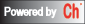# CGI-Based Numerical Calculations

 charpolycoef() Characteristic polynomial of a matrix choldecomp() Cholesky Decomposition combination() Combination xcompanionmatrix(), companionmatrix() Companion matrix condnum() Condition number of a matrix conv() Convolution conv2() Two-dimensional convolution complexsolve() Solve a complex equation corrcoef() Correlation coefficient correlation2() Two-dimensional Correlation covariance() Covariance cross() Cross product cumprod() Cumulative product calculation cumsum() Cumulative sum calculation curvefit() Fit a set of data points to a combination of specified functions deconv() Deconvolution derivative(), derivatives() Derivation cdeterminant(), determinant() Determinant of a matrix cdiagonal(), diagonal() Get a vector with diagonals of a matrix cdiagonalmatrix(),diagonalmatrix() Create a diagonal matrix difference() Differences of adjacent elements of vector dot() Dot product eigensystem() Eigenvalues and Eigenvectors expm() Matrix exponential factorial() Factorial fevalarray(), cfevalarray() Function evaluation fft() Fast Fourier Transform filter() Filter filter2() Two-dimensional Filter fminimum(), fminimums() Find the minimum value of a function fliplr() Flip matrix in left/right direction flipud() Flip matrix in up/down direction fsolve() Find zeros of a nonlinear system of equations cfunm(), funm() Matrix function evaluation fzero() Find a zero position of a nonlinear function gcd() Greatest common divisor hessdecomp() Hessenberg Decomposition identitymatrix() Identity matrix ifft() Inverse Fast Fourier Transform integral1() Integration integral2() 2D Integration integration2() 2D Integration integral3() 3D Integration integration3() 3D Integration interp1() One-dimensional interpolation interp2() Two-dimensional interpolation inverse(), cinverse() Inverse of a square matrix lcm() Least common multiple linsolve() Solve the linear equations by LU factorization llsqcovsolve() Solve the linear equations by linear squares with known covariance llsqnonnegsolve() Solve the linear equations with non-negative values by linear squares method llsqsolve() Linear Least square solution for linear equations logm() Matrix natural logarithm ludecomp() LU factorization mean(), cmean() Mean value calculation median() Median value calculation norm() Norm of a vector or matrix nullspace() Nullspace of a matrix odesolve() Ordinary Differential Equations, orthonormalbase() Orthonormal bases of a matrix pinverse() Pseudoinverse a matrix polycoef() Polynomial coefficients polyder(), polyder2() Polynomial derivative or derivative of product or quotient of two polynomials polyeval(), cpolyeval(), polyevalarray() Polynomial and its Derivative Evaluation polyevalm() Matrix polynomial function evaluation polyfit() Fit a set of data points to a polynomial function product(), cproduct() Product of all elements qrdecomp() Orthogonal-triangular QR Decomposition rank() Rank of a matrix residue() Residue rcondnum() Reciprocal condition number of a matrix roots() Roots of a polynomial rot90() Rotate matrix 90 degrees specialmatrix() Special matrix schurdecomp() Schur decomposition sort() Sorting elements sqrtm() Matrix square root std() Standard deviation sum(), csum() Sum of all elements svd() Singular value decomposition trace(), ctrace() Sum of diagonal elements of a matrix triangularmatrix(), ctriangularmatrix() Triangular matrix xcorr() Cross correlation